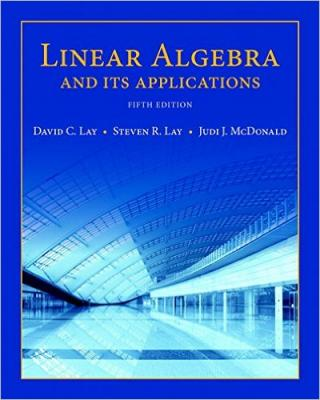### Learning goals

The main course-level goals are as follows.

A) Solve systems of linear equations.

B) Solve eigenvalue problems.

C) Analyze mathematical statements and expressions (for example, to assess whether a particular statement is accurate, or to describe solutions of systems in terms of existence and uniqueness).

D) Write logical progressions of precise mathematical statements to justify and communicate your reasoning.

E) Apply linear algebra concepts to model, solve, and analyze real-world situations.

We also have a more specific list with section-level learning goals.### Textbook

The primary reference for this course is Linear Algebra and its Applications, 5th edition, by Lay–Lay–McDonald. We will cover selections from chapters 1, 2, 3, 5, 6, and 10.

Students with a Pearson code can access the text via MyMathLab. Your lecture's course ID depends on your instructor (for example, if you are in Prof. Jankowski's lecture, the MyMathLab code is `jankowski93137`). Instructions for obtaining a Pearson code and logging into MyMathLab will be provided by your instructor. Note that this course will use WeBWorK and not MyMathLab for online homework assignments. Your MyMathLab account will give you access to an electronic version of the Lay and Stewart textb\$ problems on MyMathLab.

While the Pearson code is not truly required for this course, you are likely to need the code for other math courses you take at GaTech. In particular, Lay is bundled with other textbooks for calculus courses, so most likely buying the code now will save you money in the long run.